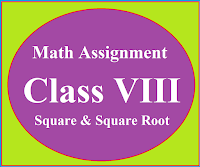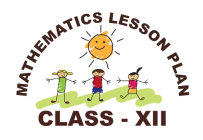### Math Assignment Class VIII | Square & Square RootMath Assignment  Class VIII | Square & Square Root Download or Print free  assignment with answer key  for   Class  8 Squares and  Square Roots.   Important and extra questions that cover all topics of square and square root and is useful and helpful for the students. Math Assignment  Class VIII | Square & Square Root LEVEL -1

### Lesson Plan Math Class 12 (Ch-2) For Mathematics Teacher

E- LESSON PLAN   SUBJECT MATHEMATICS    CLASS 10+2
Lesson Plan Class 12th Subject Mathematics for Mathematics Teacher. Effective way of Teaching Mathematics.Top planning by the teacher for effective teaching in the class.Board – CBSE CLASS –XII SUBJECT- MATHEMATICS CHAPTER 2  :-  Inverse Trigonometric Functions

TOPIC:-

Chapter 2 Inverse Trigonometric Functions.

DURATION:-

This chapter is divided into 5 modules and is completed in 10 class meetings.

PRE- REQUISITE KNOWLEDGE:-

All formulas of trigonometry  10th standard and  10+1 standard.

TEACHING AIDS:-

Green Board, Chalk,  Duster, Charts, smart board, projector, laptop etc.

METHODOLOGY:-   Lecture method

OBJECTIVES:-
• All formulas of trigonometric functions.
• Basic concepts related to the Inverse Trigonometric functions.
• Domain and range of trigonometric functions.
• Graphs of inverse trigonometric functions.
• Properties of inverse trigonometric functions.
• Some other important results without proof.

PROCEDURE :-

Start the session by checking their previous knowledge, by asking the questions related to the trigonometry class 10th and 10+1 . Now introduce the concept of trigonometry as follows.

Topic

1

Introduction

Here teacher will introduce the topic "inverse trigonometric functions" and make the discussion on all formulas of trigonometry class 10th and 10+1

Simple meanings of Inverse of a Function

sinx is a function and sin-1x  is an angle

2

Domain and Range

Here teacher will explain the domain and range of trigonometric functions and relate that with their geometric explanation

Explanation of all basic concepts of inverse trigonometric functions, with simple problems on the topic.

3

Domain and range of inverse trigonometric functions and explanation with graphs.

 Functions Domain (Value of x) Range (Value of y) Principal Value Branch [-1,1] [-1,1] R R R-(-1,1) R-(-1,1)

4

Now teacher will introduce the formulas related to the properties of inverse trigonometric functions with detail.

Other Important Results

5

Now teacher will explain the method of solving the problems based on these properties by taking some problems. Teacher may assign some questions to the students to see their progress and guide them whenever they need.

EXPECTED OUTCOMES:-
After studying this lesson students should know
• all formulas of trigonometry
• all formulas of inverse trigonometric functions.
• Students should be able to apply the different formulas in the problems
• students should understand properties of inverse trigonometric functions.
STUDENTS DELIVERABLES:-
Review questions given by the teacher.
• Students should make the presentation on the formulas of inverse trigonometric functions.
• Solve NCERT problems with examples,
• solve assignment given by the teacher.
EXTENDED LEARNING:-

Students can extend their learning in Mathematics through the RESOURCE CENTRE.  Students can also find many interesting topics on mathematics at cbsemathematics.com

ASSESSMENT TECHNIQUES:-
• Assignment sheet will be given as home work at the end of the topic.
• Separate sheets which will include questions of logical thinking and Higher order thinking skills will be given to the above average students.
• Class Test , Oral Test , worksheet and  can be made the part of assessment.
• Re-test(s) will be conducted on the basis of the performance of the students in the test.

🙏

1.2.Chal bsdk image is blur

1.Nikal madarchod paisa de phir blur hatau ga

3.good and thanks